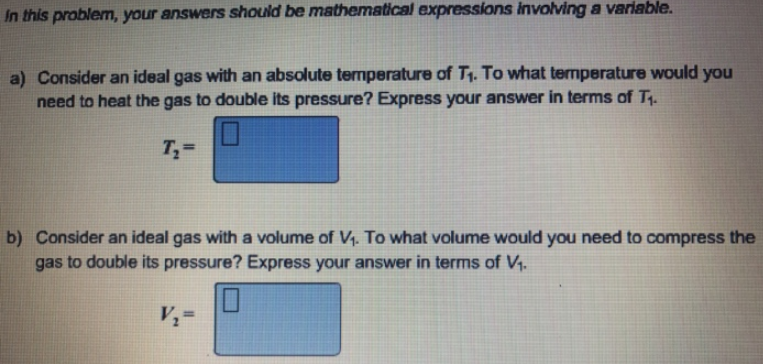# Problem: In this problem, your answers mathematical expressions involving a variable. a) Consider an ideal gas with an absolute temperature of T1. To what temperature would you need to heat the gas to double its pressure? Express your answer in terms of T1. b) Consider an ideal gas with a volume of V1. To what volume would you need to compress the gas to double its pressure? Express your answer in terms of V1.

###### FREE Expert Solution
87% (40 ratings)###### Problem Details

a) Consider an ideal gas with an absolute temperature of T1. To what temperature would you need to heat the gas to double its pressure? Express your answer in terms of T1

b) Consider an ideal gas with a volume of V1. To what volume would you need to compress the gas to double its pressure? Express your answer in terms of V1.